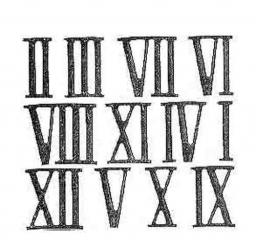# Proportion 5684

I think the number. If I reduce it three times, increase the proportion by 5/6 and decrease the sum 0.5 times, I get 2 1/7. What's the number?

c =  5/7

### Step-by-step explanation:Did you find an error or inaccuracy? Feel free to write us. Thank you!

Tips for related online calculators
Need help calculating sum, simplifying, or multiplying fractions? Try our fraction calculator.
Need help with mixed numbers? Try our mixed-number calculator.
Do you have a linear equation or system of equations and looking for its solution? Or do you have a quadratic equation?